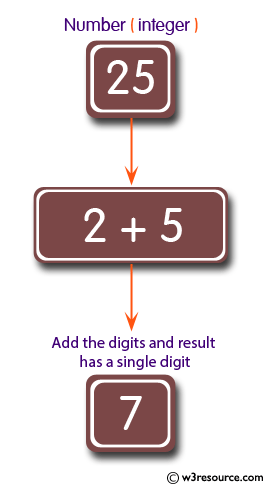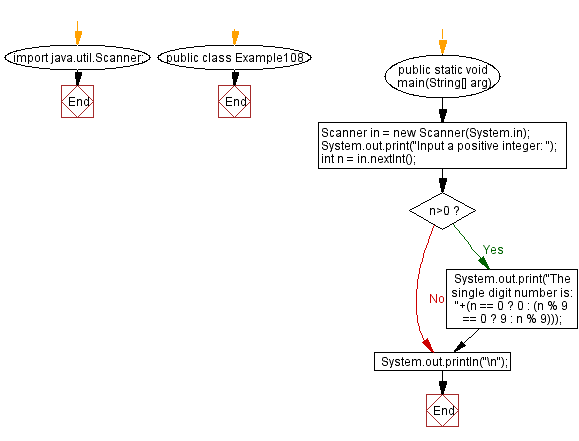﻿ Java: Add the digits of a positive integer to get one digit# Java Exercises: Add all the digits of a given positive integer until the result has a single digit

## Java Basic: Exercise-108 with Solution

Write a Java program to add all the digits of a given positive integer until the result has a single digit.

Pictorial Presentation:Sample Solution:

Java Code:

``````import java.util.Scanner;
public class Example108 {
public static void main(String[] arg) {
Scanner in = new Scanner(System.in);
System.out.print("Input a positive integer: ");
int n = in.nextInt();
if (n>0)
{
System.out.print("The single digit number is: "+(n == 0 ? 0 : (n % 9 == 0 ? 9 : n % 9)));
}
System.out.println("\n");
}
}
```
```

Sample Output:

```Input a positive integer: 25
The single digit number is: 7
```

Flowchart:Java Code Editor:

What is the difficulty level of this exercise?

Test your Programming skills with w3resource's quiz.

﻿

## Java: Tips of the Day

countOccurrences

Counts the occurrences of a value in an array.

Use Arrays.stream().filter().count() to count total number of values that equals the specified value.

```public static long countOccurrences(int[] numbers, int value) {
return Arrays.stream(numbers)
.filter(number -> number == value)
.count();
}
```

Ref: https://bit.ly/3kCAgLb